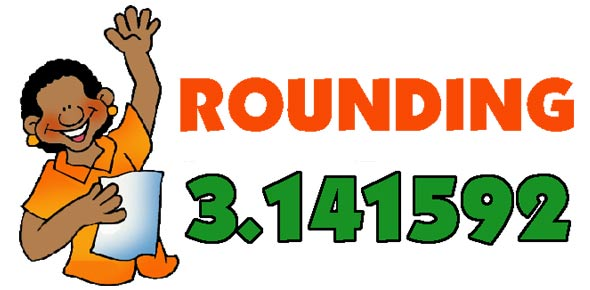# Rounding Numbers Quiz

10 Questions | Total Attempts: 7847Settings• 1.
Round the number to the nearest ten.  76
• 2.
Round the number to the nearest ten.  25
• 3.
Round the number to the nearest ten.  86
• 4.
Round the number to the nearest ten.  214
• 5.
Round the number to the nearest ten.  483
• 6.
Round the number to the nearest hundred.  361
• 7.
Round the number to the nearest hundred.  537
• 8.
Round the number to the nearest hundred.  258
• 9.
Round the number to the nearest hundred.  709
• 10.
Round the number to the nearest hundred.  455
Related TopicsBack to top